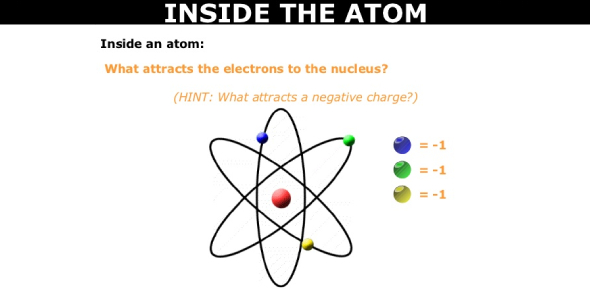# Inside The Atom

10 Questions | Total Attempts: 708SettingsFor a long time in the scientific study of chemistry, it was believed that the atom was the smallest thing in the universe. Over time, however, it was discovered that once opened there was far more to be discovered. What do you know about what can be found inside the atom? Let’s find out.

• 1.
• A.

Proton

• B.

Neutron

• C.

Electron

• D.

Nucleus

• 2.
Name the particle that has a positive charge:
• 3.
Which particle is neutral (has no charge)?
• A.

Proton

• B.

Neutron

• C.

Electron

• D.

Nucleus

• 4.
What is the atomic number of the atom shown?
• A.

4

• B.

5

• C.

6

• D.

11

• 5.
What is the atomic mass of the atom shown?
• A.

4

• B.

5

• C.

6

• D.

11

• 6.
Protons and neutrons are found where in an atom?
• 7.
Where are electrons found in an atom?
• 8.
To find the atomic number of an atom you would:
• A.

Count the protons

• B.

Count the neutrons

• C.

Count the electrons

• D.

Count the protons and neutrons

• 9.
To find the atomic mass (mass number) of an atom you would:
• A.

Count the protons

• B.

Count the electrons

• C.

Count the neutrons

• D.

Count the protons and neutrons

• 10.
Which particle has a negative charge?
Related TopicsBack to top